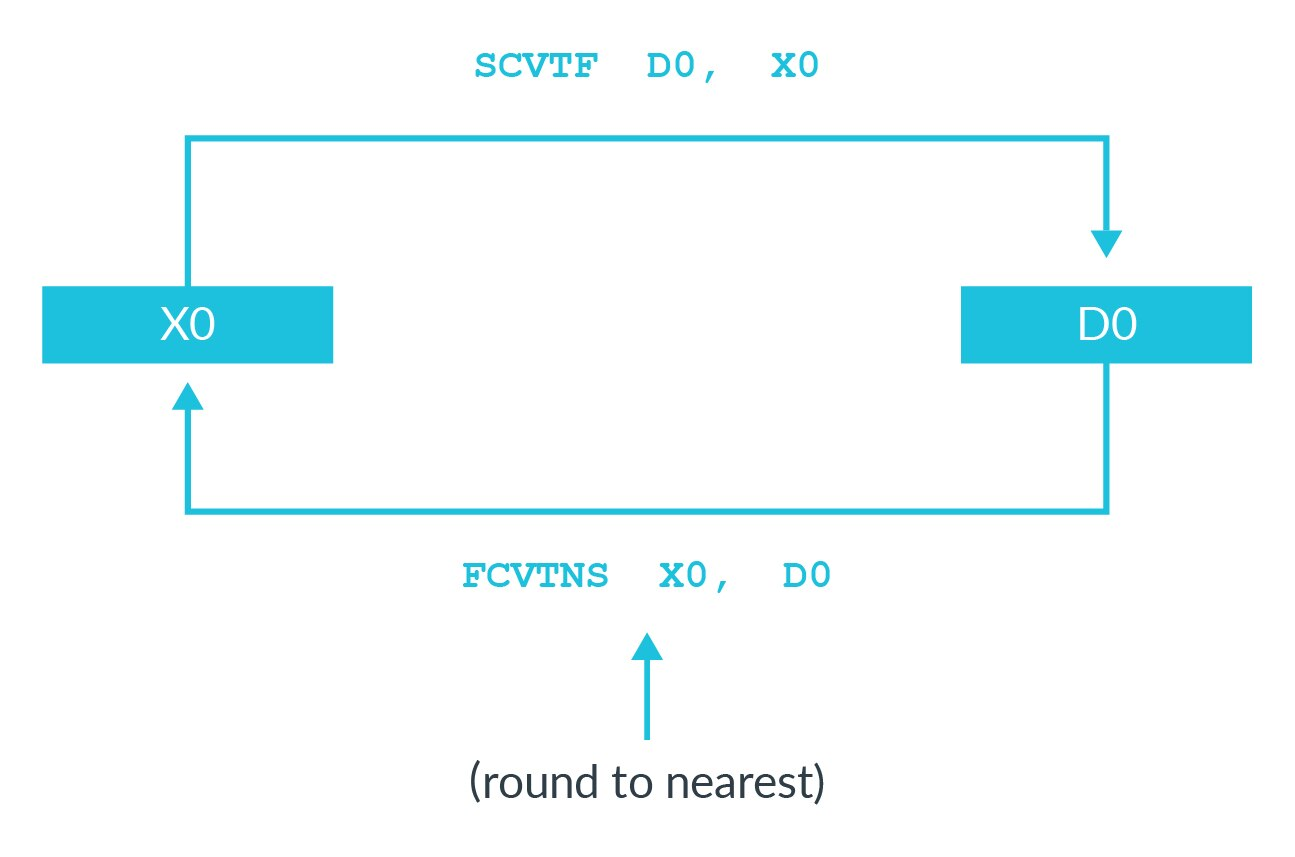## Format conversion

We have seen that the `MOV` and `MVN` instructions copy the value from one register to another. Similarly, `FMOV` can be used to copy between floating-point and general purpose registers.

However, using `FMOV` copies the literal bit pattern between the registers. There are also instructions that can convert to the closest representation, as this figure shows:In this example, imagine that `X0` contains the value 2 (positive integer 2):

```X0 = 0x0000_0000_0000_0002
```

Then, the following sequence is executed:

```FMOV D0, X0

SCVTF D1, X0
```

Both instructions “copy” X0 into a D register. However, the results are quite different:

```D0 = 0x0000_0000_0000_0002 = 9.88131e-324
D1 = 0x4000_0000_0000_0002 = 2.0
```

The `FMOV` copied the literal bit pattern, which is a very different value when interpreted as a floating-point value. The `SCVTF` converted the value in `X0` to the closest equivalent in floating-point.

Similarly, `FCTxx` can be used to convert a floating-point value to its closest integer representation. In this instance, different values of '`xx`' control the rounding mode used.